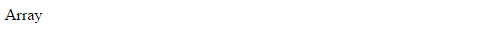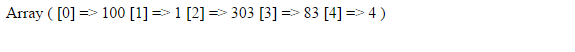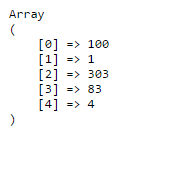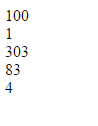learn

category: Basics

category: Basics

# Dealing with Arrays: Lesson 1

Arrays are probrably one of the most important feature in every programming/scripting language.
Today we will cover some of the arrays basics.

What is an array?
By definition, an array is a data structure that stores one or more similar (or different) type of values in a single value (variable).
For example if you want to store 13 integers, 24 strings, and 1000 floating numbers then instead of defining 1037 variables its easy to define an array of 1037 length.

How many types of arrays are there?
There are 3 different types of arrays, each of them can be accessed using the array index (hold your horses, we will get to that in a few)

1. Numeric Array: this type of array comes with a numeric index (0, 2, 3, 4, ...., 4000, etc). Every value is stored in a unique block, each block has its own unique key (index) number.
2. Multidiemnsional Array: the name explains itself, an array containing one or more arrays. Similar to the numeric array in concept, multi-dimensional array can store numbers, strings, and arrays. Each array stored in the multidimensional array can store even more multidimensional arrays. Each value can be accessed by means of mutliple indices (we will speak more of this in another lesson).
3. Associative Array: similar to the numeric array, except that the values can be accessed either by their index key or by a defined string ( has to be unique as well).

Now that we have defined the array and discussed its 3 types, lets get to the real thing: coding!

Unlike other languages (like C++ for example), in PHP initializing an array does not require the programmer to define its size. You simply initialize it with: array();

In the first example we told PHP that \$first_array will hold an array of data by simply using array().
Lets add some data to our array and run our code using 'echo' to see what will be displayedAs shown in Fig-1, we can only see the word "Array" - that is because you cannot echo an array!
There are several methods to show its data, lets use a built-in function called print_r() to see what we have in our arrayWe will add the html "pre" tag before print_r() to display the results nicelyLooks better!
As you can see, the data we added to our array are displayed in order; but what do the numbers in the subscript [] stand for?
Those are the "indices" we talked about (the unique keys). Each element in the array has its own unique identifier that we call "index".
Okay, but why do we need an index? How do we use them?

We use the indices to access the element we want. Say we want to display the 4th element (83), we will simply tell PHP to grab the -3rd- index in the array.
3rd index? But we thought we were going to access the 4th element!
Yes, the 4th element in the array is located at the 3rd position because the array starts with "0" as its first index (unless defined otherwise).
So, an array of two elements will have the following indices: 0 and 1
An array of 3 elements will have 0, 1, and 2 as its indices
An Array of 1000 elements will have 0, 1, 2, 3, ...., 999 as its indecies
An array of n elements will have 0, 1, 2, 3, ..., n-1 as its indecies.

Now we will display the 4th element in the array, we can simply do that by echoing it.

83 is displayed correctly.
We use the subscript [] to access a specific index in an array.
To access the first element, we simply replace 3 with 0
echo \$first_array;

What if we want to display each element in the array, and each element has to be in a new line?
Easy, we will loop through the array using any loop method (do while, for, or while). But first, we need to know the size of the array.
Our array consists of 5 elements, so its size is 5.You noticed that we started \$i with a 0. Why? Because the first index of the array is 0
But why we stopped at \$i < 5 not \$i <= 5? Because an array of 5 elements stops at index #4. If we replace < with <=, PHP will try to access \$first_array (the fifth index that does not exist) and will throw a "undefined offset: 5" notice.